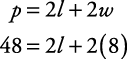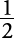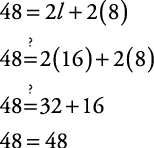## Formulas

A formula is an equation that describes a relationship between unknown values. Many problems are solved easily if the correct formula is known. To use formulas to solve problems, perform the following steps:

1. Identify the appropriate formula.

2. Replace the variables in the formula with their known values.

3. Solve the formula for the remaining variable(s).

4. Check the solution in the original problem.

5. State the solution.

##### Example 1

Find the length of a rectangle (see Figure 1) if its perimeter is 48 inches and its width is 8 inches.

An appropriate formula is p = 2 l + 2 w, where p represents the perimeter, l the length, and w the width of the rectangle. Replace each variable with its known value.Now, solve for the remaining variable.

48 = 2 l + 16

32 = 2 l

Multiply each side by(or divide each side by 2).

16 = l

Check with the original problem.The length of the rectangle is 16 inches.